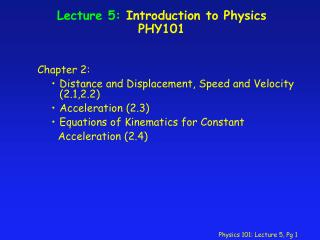DownloadDownload PresentationLecture 5: Introduction to Physics PHY101

# Lecture 5: Introduction to Physics PHY101

Télécharger la présentation## Lecture 5: Introduction to Physics PHY101

- - - - - - - - - - - - - - - - - - - - - - - - - - - E N D - - - - - - - - - - - - - - - - - - - - - - - - - - -
##### Presentation Transcript

1. Lecture 5: Introduction to Physics PHY101 Chapter 2: • Distance and Displacement, Speed and Velocity (2.1,2.2) • Acceleration (2.3) • Equations of Kinematics for Constant Acceleration (2.4)

2. Displacement and Distance • Displacement is the vector that points from a body’s initial position, x0, to its final position, x. The length of the displacement vector is equal to the shortest distance between the two positions. x = x –x0 Note: The length of xis (in general) not the same as distance traveled !

3. Average Speed and Velocity • Average speed is a measure of how fast an object moves on average: average speed = distance/elapsed time Average speed does not take into account the direction of motion from the initial and final position.

4. Average Speed and Velocity • Average velocity describes how the displacement of an object changes over time: average velocity = displacement/elapsed time vav = (x-x0) / (t-t0) = x/ t Average velocity also takes into account the direction of motion. Note: The magnitude of vav is (in general) not the same as the average speed !

5. Instantaneous Velocity and Speed • Average velocity and speed do not convey any information about how fast the object moves at a specific point in time. • The velocity at an instant can be obtained from the average velocity by considering smaller and smaller time intervals, i.e. Instantaneous velocity: v = lim  t-> 0x/ t Instantaneous speed is the magnitude of v.

6. Concept Question • If the average velocity of a car during a trip along a straight road is positive, is it possible for the instantaneous velocity at some time during the trip to be negative? 1 - Yes 2 - No correct If the driver has to put the car in reverse and back up some time during the trip, then the car has a negative velocity. However, since the car travels a distance from home in a certain amount of time, the average velocity will be positive.

7. Acceleration • Average acceleration describes how the velocity of an object moving from the initial position to the final position changes on average over time: aav = (v-v0) / (t-t0) = v/ t • The acceleration at an instant can be obtained from the average acceleration by considering smaller and smaller time intervals, i.e. Instantaneous acceleration: a = lim  t-> 0v/ t

8. Concept Question • If the velocity of some object is not zero, can its acceleration ever be zero ? 1 - Yes 2 - No correct If the object is moving at a constant velocity, then the acceleration is zero.

9. Concept Question • Is it possible for an object to have a positive velocity at the same time as it has a negative acceleration? 1 - Yes 2 – No correct An object, like a car, can be moving forward giving it a positive velocity, but then brake, causing deccelaration which is negative.

10. Kinematics in One DimensionConstant Acceleration • Simplifications: In one dimension all vectors in the previous equations can be replaced by their scalar component along one axis. For motion with constant acceleration, average and instantaneous acceleration are equal. For motion with constant acceleration, the rate with which velocity changes is constant, i.e. does not change over time. The average velocity is then simply given as vav = (v0 +v)/2

11. Kinematics in One DimensionConstant Acceleration Consider an object which moves from the initial position x0, at time t0 with velocity v0, with constant acceleration along a straight line. How does displacement and velocity of this object change with time ? a = (v-v0) / (t-t0) => v(t) = v0 + a (t-t0) (1) vav = (x-x0) / (t-t0) = (v+v0)/2 => x(t) = x0 + (t-t0) (v+v0)/2 (2) Use Eq. (1) to replace v in Eq.(2): x(t) = x0 + (t-t0) v0 + a/2 (t-t0) 2 (3) Use Eq. (1) to replace (t-t0) in Eq.(2): v2 = v02 + 2 a (x-x0 ) (4)

12. Summary of Concepts • kinematics: A description of motion • position: your coordinates • displacement: x = change of position • velocity: rate of change of position • average : x/t • instantaneous: slope of x vs. t • acceleration: rate of change of velocity • average: v/t • instantaneous: slope of v vs. t